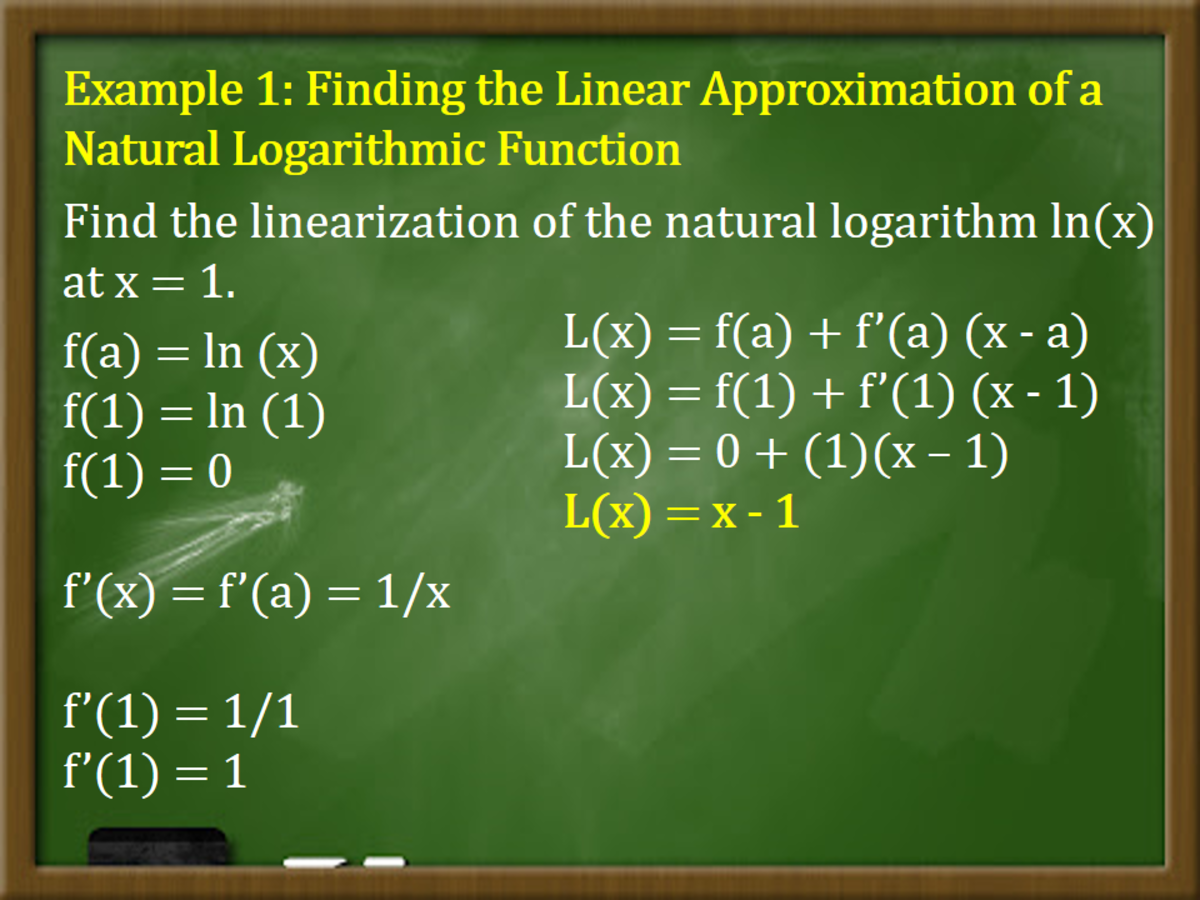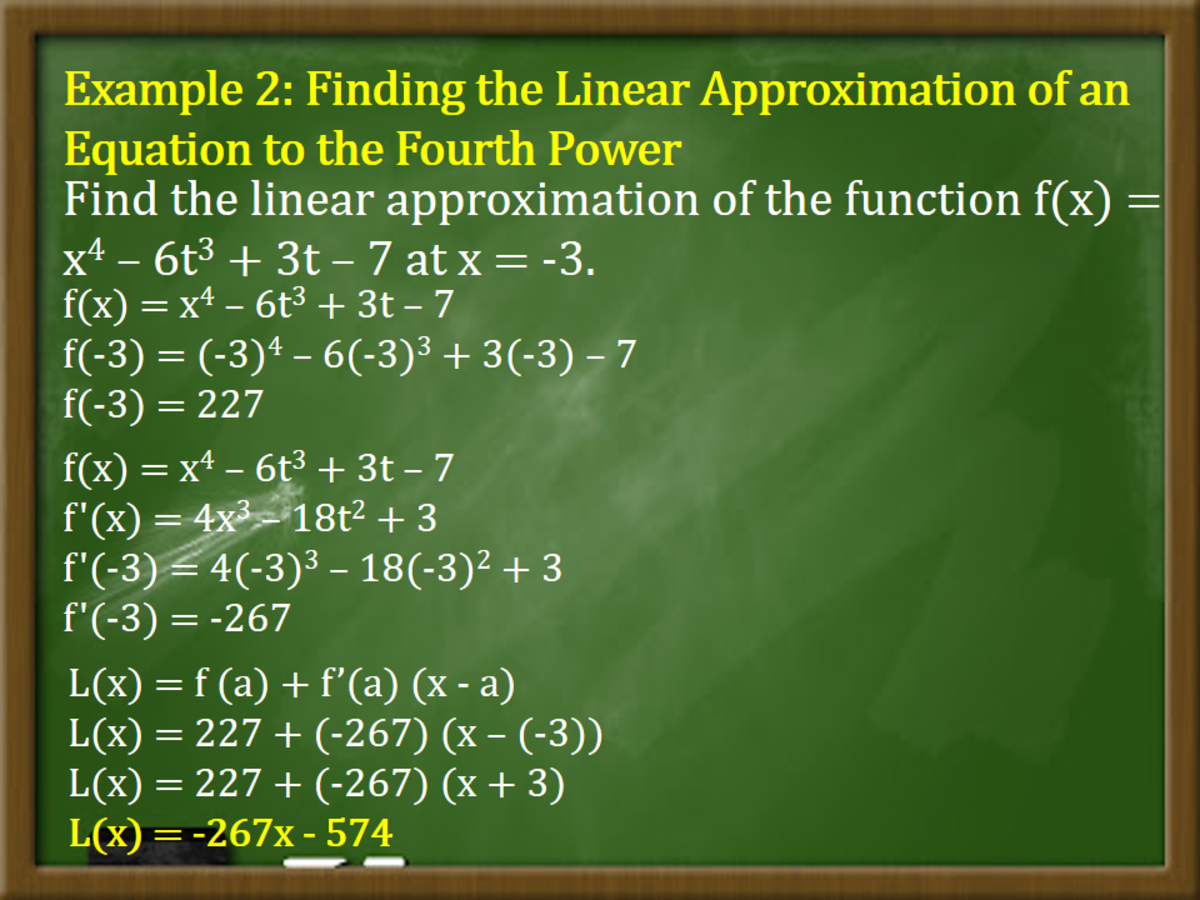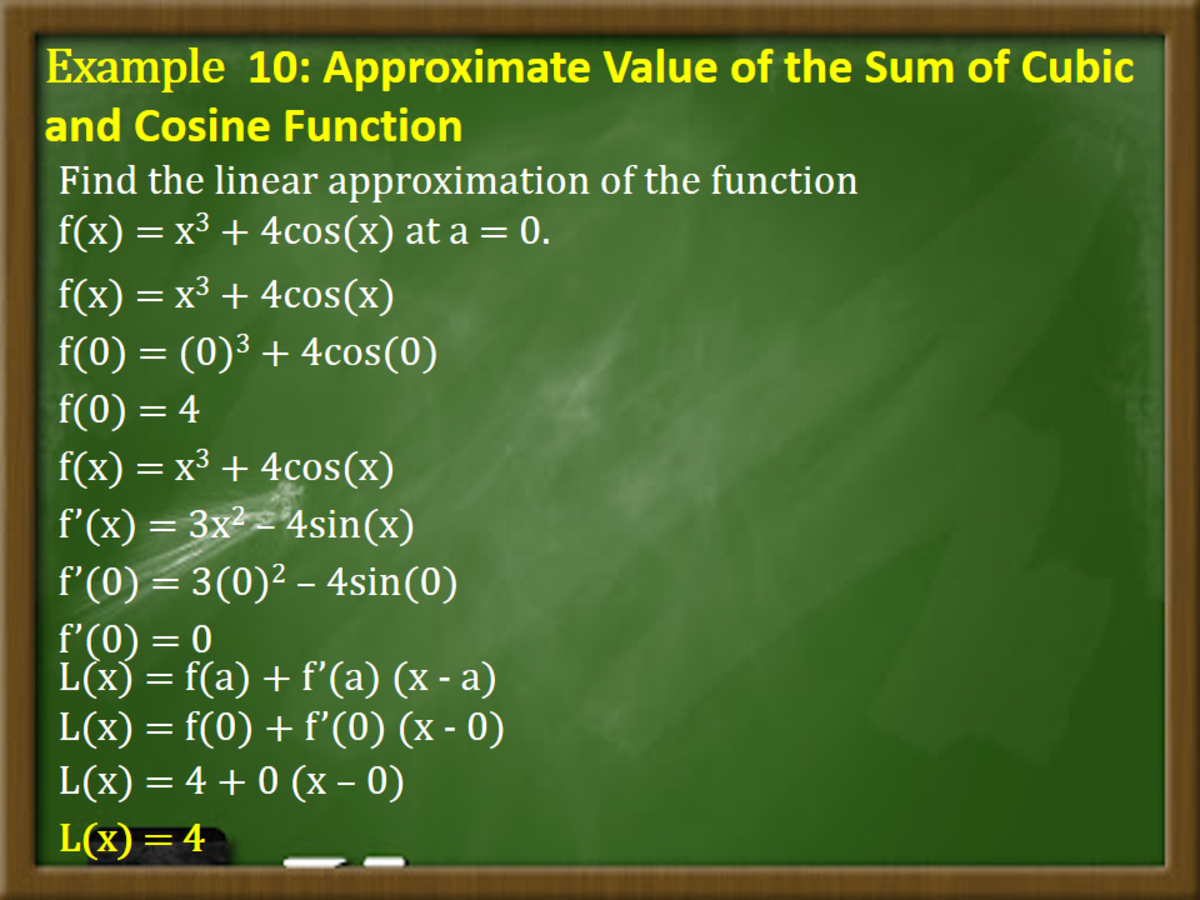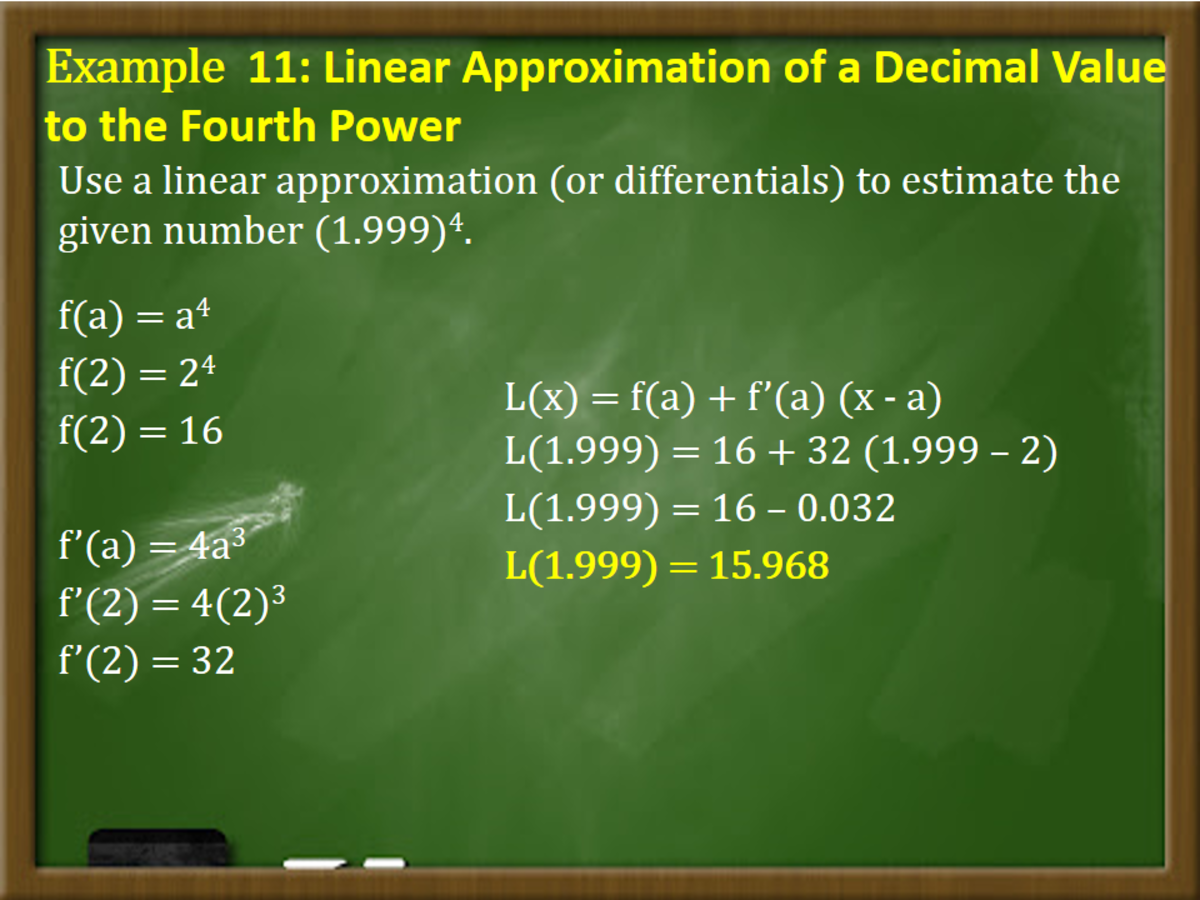# Linear Approximation and Differentials in Calculus

• Author:
• Updated date:

Ray is a licensed engineer in the Philippines. He loves to write about mathematics and civil engineering.

Linear Approximation, or sometimes referred to as the Linearization or Tangent Line Approximation, is a calculus method that uses the tangent line to approximate another point on a curve. Linear Approximation is an excellent method to estimate f (x) values as long as it is near x = a.

The given figure below shows a curve that lies very close to its tangent line near the point of tangency. In fact, by zooming in toward an end on a differentiable function graph, the graph looks more and more like its tangent line. This method is the basis for a way of finding approximate values of a function.

The idea is that it might be easy to calculate a value of f (a) of a function but challenging to compute nearby values of the function f. So we settle for the easily calculated values of the linear function L, whose graph is the tangent line of the function f at (a, f (a)).

### Linear Approximation Formula

Use the tangent line at (a, f (a)) as an approximation to the curve y=f(x) when x is near a. The tangent line equation is shown below.

y = f (a) + f’ (a) (x-a)

The Approximation given by the equation below is called the linear Approximation or tangent line approximation of f at a. In calculus, a linear approximation is an approximation of a general equation using a linear function.

f (x) = f (a) + f’ (a) (x-a)

The linear function whose graph is this tangent line, shown below, is called the Linearization of the function f at a.

L (x) = f (a) + f’ (a) (x - a) [equation of tangent line]

## How to Perform Linear Approximation

There are three easy steps on how to perform Linear Approximation.

1. Plug in the given value of the given variable a for x, then solve for the value of y to find the ordered pair.
2. Take the derivative of the given function f to find the slope of the tangent line f'.
3. Next, there are two options you can go through in finding the Linearization of the given function.

Option 1: Plug in the ordered pair from the first step and solve for the slope m or dy/dx. The Linearization is found by substituting the ordered pair and slope obtained from the previous actions into a point-slope equation.

y – y1 = m (x – x1)

Option 2: Use the given formula of the equation of the tangent line in finding the Linearization.

Scroll to Continue

L (x) = f (a) + f’ (a) (x - a)

## Example 1: Finding the Linear Approximation of a Natural Logarithmic Function

Find the linearization of the natural logarithm ln(x) at x = 1.

Solution

Consider the natural logarithm function ln(x). Calculate the derivative of the function and its value at point a.

f(a) = ln (x)

f(1) = ln (1)

f(1) = 0

f’(x) = f’(a) = 1/x

f’(1) = 1/1

f’(1) = 1

In getting the linearization of the given function, use the linear approximation function L(x).

L(x) = f(a) + f’(a) (x - a)

L(x) = f(1) + f’(1) (x - 1)

L(x) = 0 + (1)(x – 1)

L(x) = x - 1

Therefore, the linearization of the given natural logarithm is L(x) = x – 1.Finding the Linear Approximation of a Natural Logarithmic FunctionJohn Ray Cuevas

## Example 2: Finding the Linear Approximation of an Equation to the Fourth Power

Find the linear approximation of the function f(x) = x4 – 6t3 + 3t – 7 at x = -3.

Solution

First, substitute the value of x = -3 to the given equation.

f(x) = x4 – 6t3 + 3t – 7

f(-3) = (-3)4 – 6(-3)3 + 3(-3) – 7

f(-3) = 227

Get the derivative of the given equation and substitute the value of x = -3.

f(x) = x4 – 6t3 + 3t – 7

f'(x) = 4x3 – 18t2 + 3

f'(-3) = 4(-3)3 – 18(-3)2 + 3

f'(-3) = -267

Substitute the values of f(-3) and f'(-3) to the linearization formula of a function.

L(x) = f (a) + f’(a) (x - a)

L(x) = 227 + (-267) (x – (-3))

L(x) = 227 + (-267) (x + 3)

L(x) = -267x - 574

The linear approximation of the function f(x) = x4 – 6t3 + 3t – 7 at x = -3 is L(x) = -267x – 574.Finding the Linear Approximation of an Equation to the Fourth PowerJohn Ray Cuevas

## Example 3: Finding the Linearization of a Square Root Function

Find the linearization of the function f(x) = √x + 3 at a = 1 and use it to approximate the numbers √3.98 and √4.1. Are these approximations overestimates or underestimates?

Solution

Find the derivative of the function f(x) = (x + 3)1/2.
f(x) = (x + 3)1/2
f'(x) = (1/2) (x + 3)-1/2
f'(x) = 1 / (2√(x + 3))

Substitute a = 1 to the equation and the derivative of the function
f(1) = (1 + 3)1/2
f(1) = 2
f'(1) = 1 / (2√(1 + 3))
f'(1) = ¼
Put the obtained values of f (1) and f' (1) to the equation of the tangent line. Therefore, the linearization is given below.
L(x) = f (1) + f’(1) (x - 1)
L(x) = 2 + (1/4) (x – 1)
L(x) = (7/4) + (x/4)

After solving the linearization of the given square root function, approximate the value of the given square root numbers when x is near 1. As seen from the graph, the approximations are overestimated since the tangent line lies above the curve.
√3.98 = (7/4) + (x/4)
√3.98 = (7/4) + (0.98/4)
√3.98 = 1.995
√4.1 = (7/4) + (x/4)
√4.1 = (7/4) + (1.1/4)
√4.1 = 2.025

The table below shows and compare the estimates from the linear approximation with real values. According to the graph, the tangent line approximation gives a reliable estimate when x is close to 1, but the approximate linear accuracy decreases when x moves farther away from 1.

Square Root EquationsxL(x) EstimatesActual Value

√3.9

0.9

1.975

1.97484176…

√3.98

0.98

1.995

1.99499373…

√4

1

2

2.00000000…

√4.05

1.05

2.0125

2.012462117…

√4.1

1.1

2.025

2.02484567…

√5

2

2.25

2.23606797…

√6

3

2.5

2.44948974…

The corresponding linear approximation of the function (x + 3)1/2 is equal to (7/4) + (x/4). Also, the overestimated approximate values of √3.98 and √4.1 are 1.995 and 2.025, respectively.

## Example 4: Finding the Linear Approximation of √x

Find the linear approximate of the square root function f(x) = √x at x = 16. Then, use the approximation to estimate √16.1.

Solution

Solve for the value of the given function for f (16) and f' (16).

f(x) = √x

f(16) = √16

f(16) = 4

f'(x) = √x

f'(16) = 1/(2√x)

f'(16) = 1/(2√16)

f'(16) = 1/(2√x)

f'(16) = 1/8

Substitute the obtained values to the linearization formula.

L(x) = f (16) + f’ (16) (x - 16)

L(x) = 4 + (1/8) (x – 16)

Using the equation obtained, use the linear approximation to estimate √16.1.

L(16.1) = 4 + (1/8) (16.1 – 16)

L(16.1) = 4.0125

The linear approximate of the function f(x) = √x at x = 16 is L (x) = 4 + (1/8) (x – 16). Using this, the estimated value of √16.1 is 4.0125.

## Example 5: Predicting a Temperature From a Linear Approximation

Suppose that after you stuff a chicken, its temperature is ten degrees Celsius, you put it in a 165°C oven. After an hour, the meat thermometer indicates that the chicken's temperature is 34°C, and after two hours, it displays 54°C. Predict the temperature of the chicken after three hours.

Solution

The function T (t) represents the temperature of the chicken after t hours. Given the temperature of the chicken in different conditions, then T (0) = 10, T (1) = 34, and T (2) = 54. To make a linear approximation with a = 2, then estimate the derivative of T at 2.

T'(2) = limx→2 [T (t) – T (2)] / (t – 2)

Use the difference quotient with t = 1 in estimating the value of T' (2).

T'(2) = [T (1) – T (2)] / (1 – 2)

T'(2) = [34 – 54] / (-1)

T'(2) = 20

The temperature change between t = 1 and t = 2 is 20°C/h. With the obtained estimate, the linear approximation for the temperature after three hours is given below.

T(3) = T(2) + T’(2) (3 - 2)

T(3) = 54 + 20 (1)

T(3) = 74

The predicted temperature of the chicken after three hours is 74°C.

## Example 6: Linear Approximation of a Sine Function

Find the linear approximation for sin (θ) at θ = 0.

Solution

Compute the tangent line equation to the given equation sin (θ) at θ = 0.

f(θ) = sin(θ)

f(0) = 0

f'(θ) = cos(θ)

f'(0) = 1

Compute the linear approximation by substituting the formula for the tangent line equation.

L(x) = f(a) + f’(a) (x-a)

L(θ) = f(0) + f'(0) (θ – 0)

L(θ) = 0 + 1(θ)

L(θ) = θ

Therefore, sin (θ) = θ considering θ stays small.

## Example 7: Linear Approximation of Roots and Powers

Find the linear approximation of f(x) = (1 + x)n at x = 0. Use the resulted linear approximation to estimate (1.05)5.

Solution

Given the function f(x) = (1 + x)n, solve for f(x) and f’(x) at x = 0.

f(x) = (1 + x)n

f(0) = (1 + 0)n

f(0) = 1

f’(x) = n(1 + x)n-1

f’(0) = n(1 + 0)n-1

f’(0) = n

In getting the approximate value of f(x) = (1 + x)n, apply the approximate formula or the tangent line equation.

L(x) = f(a) + f’(a) (x - a)

L(x) = f(0) + f’(0) (x – 0)

L(x) = 1 + nx

Next, evaluate (1.05)5 by evaluating L(1.05) when n = 5.

L(0.05) = 1 + (0.05)(5)

L(0.05) = 1 + 0.25

L(0.05) = 1.25

Therefore, the estimated value of (1.05)5 is 1.25.

## Example 8: Linear Approximation of a Cos(X)

Find the approximate value for the function f(x) = cos (x) at x = π/2 using the linear approximation formula. Use the result to approximate the value of cos(89π/180).

Solution

Compute the tangent line equation to the given equation cos(x) at x = π/2.

f(x) = cos(x)

f(π/2) = cos(π/2)

f(π/2) = 0

f'(x) = -sin(x)

f'(π/2) = -sin(π/2)

f'(π/2) = -1

Compute the linear approximation by substituting the formula for the tangent line equation.

L(x) = f(a) + f’(a) (x-a)

L(x) = f(π/2) + f'(π/2) (x – π/2)

L(x) = 0 – 1(x - π/2)

L(x) = -x + π/2

Substitute x = π/180 to the linearization equation to solve the value of cosine (π).

L(x) = -x + π/2

L(89π/180) = -(89π/180) + π/2

L(89π/180) = π/180 = 0.0175

The approximate value of the function f(x) = cos (89π/180) is π/180 or approximately 0.0175.

## Example 9: Approximate Value of a Square Root Function

Find the approximate value for the square root function f(x) = √50 using the linear approximation formula.

Solution

The function can be read as f(x) = √x at x = 50. Find the value of the function and its derivative at a = 49.

f(a) = √x

f(a) = √49

f’(a) = (√a)’ = 1 / (2√a)

f’(49) = 1 / (2√a)

f’(49) = 1 / (2√49)

f’(49) = 1 / 14

Using the tangent line equation, compute the approximate value at x = 50.

L(x) = f(a) + f’(a) (x - a)

L(x) = √49 + (1/14) (50 – 49)

L(50) = 7 + (1/14)

L(50) = 99/14

L(50) = 7.0714

The approximate value of the function f(x) = √50 is 7.0174.

## Example 10: Approximate Value of the Sum of Cubic and Cosine Function

Find the linear approximation of the function f(x) = x3 + 4cos(x) at a = 0.

Solution

Solve for the value of the given cosine function for f(0) and f'(0). Solve for the value of the function and its derivative at a = 0.

f(x) = x3 + 4cos(x)

f(0) = (0)3 + 4cos(0)

f(0) = 4

f(x) = x3 + 4cos(x)

f’(x) = 3x2 – 4sin(x)

f’(0) = 3(0)2 – 4sin(0)

f’(0) = 0

Substitute the value of f(0) and f’(0) to the tangent line equation.

L(x) = f(a) + f’(a) (x - a)

L(x) = f(0) + f’(0) (x - 0)

L(x) = 4 + 0 (x – 0)

L(x) = 4

The linear approximation of the given function is the horizontal line L(x) = 4.Example 10: Approximate Value of the Sum of Cubic and Cosine FunctionJohn Ray Cuevas

## Example 11: Linear Approximation of a Decimal Value to the Fourth Power

Use a linear approximation (or differentials) to estimate the given number (1.999)4.

Solution

Consider the given function (1.999)4 as x4. Therefore, find the f(a) and f’(a) at a=2 since it is the nearest possible integer.

f(a) = a4

f(2) = 24

f(2) = 16

f’(a) = 4a3

f’(2) = 4(2)3

f’(2) = 32

Substitute the value of f(2) and f’(2) to the tangent line equation.

L(x) = f(a) + f’(a) (x - a)

L(1.999) = 16 + 32 (1.999 – 2)

L(1.999) = 16 – 0.032

L(1.999) = 15.968

The approximate value of (1.999)4 is line 15.968.Linear Approximation of a Decimal Value to the Fourth PowerJohn Ray Cuevas

## Example 12: Linear Approximation of a Natural Logarithmic Equation

Use linear approximation to estimate the value of e0.2.

Solution

Let f(x) = ex and set a = 0 in solving for f(a) and f’(a).

f(a) = ea

f(0) = e0

f(0) = 1

f’(a) = ea

f(0) = e0

f(0) = 1

In getting the approximate value of e0.2, apply the approximate formula or the tangent line equation.

L(x) = f(a) + f’(a) (x - a)

L(0.2) = f(0) + f’(0) (0.2 - 0)

L(0.2) = 1 + 1 (0.2 – 0)

L(0.2) = 1.2

Therefore, the approximate value of e0.2 is 1.20.

## Example 13: Approximate Value of Inverse Trigonometric Function

Use a linear approximation (or differentials) to estimate the inverse trigonometric equation y = arcos (0.52).

Solution

Suppose that f(x) = arcos (x) and a = 0.5. Replace the increment of the given function ∆y by its differential and compute the inverse trigonometric function's approximate value.

f(a) = arcos (a)

f(0.5) = arcos (0.5)

f(a) = arcos (a)

f’(a) = -1/√(1-x2)

f’(0.5) = -1/√(1-0.52)

f’(0.5) = -1/√0.75

f’(0.5) = -1.1547

In getting the approximate value of y = arcos (0.52), apply the approximate formula or the tangent line equation.

L(x) = f(a) + f’(a) (x - a)

L(0.52) = f(0.5) + f’(0.5) (0.52-0.5)

L(0.52) = arcos (0.5) – 1.1547 (0.52-0.5)

L(0.52) = 1.0472 – 0.0231

L(0.52) = 1.0241

The approximate value of the function y = arcos (0.52) is 1.0241.

## Example 14: Approximate Value of a Linear Function

Find the linear approximation of the function g(x) = 51 + 3x at x = 0.

Solution

Solve for the value of the given linear function for g(0) and g'(0). Solve for the value of the function and its derivative at a = 0.

g(a) = 51 + 3(0)

g(0) = 51 + 0

g(0) = 51

g’(a) = 51 + 3x

g’(0) = 0 + 3

g’(0) = 3

In getting the approximate value of g(x) = 51 + x, apply the approximate formula or the tangent line equation.

L(x) = f(a) + f’(a) (x - a)

L(x) = g(a) + g’(a) (x - a)

L(x) = g(0) + g’(0) (x - 0)

L(x) = 51 + 1(0 – 0)

L(x) = 51

Therefore, the approximate value of the given function g(x) = 51 + 3x at x = 0 is 51.

## Example 15: Finding the Approximate Value of a Quadratic Function

Given the function f(x) = 5 / x2, use linear approximation at a = 1 to estimate the value of f(0.99).

Solution

Solve for the value of the given linear function for f(x) and f'(x) when x = 0.99. Solve for the value of the function and its derivative at a = 1.

f(x) = 5 / x2

f(a) = 5 / a2

f(1) = 5 / 12

f(1) = 5

f(x) = 5 / x2

f’(x) = 5x-2

f’(1) = 5(-2) a-2-1

f’(1) = -10a-3

f’(1) = -10/a3

f’(1) = -10/(1)3

f’(1) = -10

In getting the approximate value of f(x) = 5 / x2, apply the approximate formula or the tangent line equation.

L(x) = f(a) + f’(a) (x - a)

L(0.99) = f(1) + f’(1) (0.99 – 1)

L(0.99) = 5 - 10 (0.99 - 1)

L(0.99) = 5 + 0.1

L(0.99) = 5.10